# Bleeder Resistor: What is it, and Why is it Used?

Contents

## What is a Bleeder Resistor?

A bleeder resistor is a standard resistor connected in parallel with the output of a high-voltage power supply circuit for the purpose of discharging the electric charge stored in the power supply’s filter capacitors when the equipment is turned OFF. This is done for safety reasons.

If someone accidentally touches during the service of equipment in the OFF state, there may be a chance to get shocked even if the device is in OFF condition. Hence, it is necessary to discharge the capacitor for safety purposes. The bleeder resistor is hence used to help prevent unwanted electrical discharges.

## Importance of Bleeder Resistor in a Circuit

To know the importance of a bleeder resistor, we need a circuit that used a filter. For example, we choose a full-wave rectifier circuit. The output of the rectifier is not pure DC signal. It is pulsating DC signal and this supply cannot directly give to the load.

So, we use a filter circuit to make the output of the rectifier pure DC signal. And the filter consists of the capacitors and inductors. The below circuit shows that the output of the rectifier is given to the load via the filter circuit and bleeder resistor.

As shown in the above figure, the bleeder resistor is connected in parallel with the capacitor. The capacitor is charged at peak value during the device is in ON condition. And if we turn OFF the device, some amount of charge is still saved by that capacitor.

Now if the bleeder resistor is not connected and someone touched the terminals, the capacitor will discharge through that person. And that person will get shocked.

But if we connect a standard resistor in parallel with that capacitor, the capacitor will discharge through the resistor.

## How to Choose a Bleeder Resistor

If you select a small valued resistor, it will provide high-speed bleeding. But it consumes more power. And if you select a high valued resistor, it will cause less power loss but bleeding speed is less.

So, the designer has to select a properly valued resistor that is high enough to not interfere with the power supply and low enough to discharge the capacitor in a short time.

To calculate the appropriate value of the bleeder resistor, consider the relationship among the momentary voltage across capacitor Vt, the bleeder resistor (R), and the initial value Vu. The total capacitance is C and the momentary period is t. Then you can calculate the value of bleeder resistance from the below equation.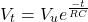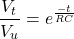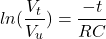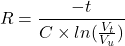In the above equation, keep the value of momentary voltage low for safety purposes. But if you make it zero, the time required for the bleeder resistor to discharge capacitor is infinite. Hence the designer has to put the proper value of safe voltage and time required to discharge a capacitor.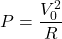Now if you select a value of bleeder resistor for fast discharge, resistance will very low. And it will increase the power loss. In the above equation, V0 is the initial voltage, and P is the power consumed by the bleeder resistance.

Hence, the designer has to decide the desired value for the power loss and bleeding speed of resistor.

## Applications of Bleeder Resistors

The bleeder resistor is used in the circuit for safety purposes. But it is also useful to improve the voltage regulation and a tapped resistor used as a voltage divider.

### Voltage Regulation

The voltage regulation is defined as the ratio of the difference between no-load and full-load voltage to no-load voltage. The equation of voltage regulation is as shown in the below equation.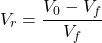Where V0 is no-load voltage and Vf is a full-load voltage. For good voltage regulation, the value of Vr is kept as low as possible.

Now if the bleeder resistor is connected in parallel with a filter capacitor and load resistor. Therefore, the voltage drop occurs in load resistance as well as in bleeder resistance.

When supply is given, the voltage drop across the load resistance comes into the picture. But, when supply is OFF, the voltage drop across the bleeder resistance comes into the picture. Hence, it increases the value of the no-load voltage.

Therefore, it will decrease the value of the difference of no-load voltage and full-load voltage that improves the voltage regulation of the circuit.

### Voltage Division

If you want multiple voltage arrangements in your circuit, a tapped resistor is used as a bleeder resistor. The circuit arrangement is as shown in the below figure.

As shown in the above figure, the bleeder resistor has three tapings. By using proper tapping, we can have three different voltage across the load. Therefore, this provides a function of a voltage divider.

### Safety Purposes

The main function of the bleeder resistor is safety. It is used to discharge the capacitor after the device is turned OFF. The circuit arrangement for this is as shown in the below figure.

The filter consists of inductors and capacitors. The bleeder resistors are used to connect it with parallel to the filter capacitor. Once the device is turned OFF, some amount of charge is stored inside the capacitor.

This charged capacitor may discharge through the person. And that person may get shocked. Hence, a bleeder resistor is used to connect in parallel with the capacitor to discharge it after the device is turned OFF.

Want To Learn Faster? 🎓
Get electrical articles delivered to your inbox every week.
No credit card required—it’s 100% free.